# 分类刷算法题 （五） 二叉树 | 前端er• 今天来刷二叉树，我们只做一些比较经典面试常考的二叉树题目，不去纠结难题

## 一、二叉树经典题目

• 104. 二叉树的最大深度 - 力扣（LeetCode）
• 难度：简单
• 这个太经典啦，但是代码数量也很少，送分题好嘛
• 思路：
• 使用递归，每次返回左右子树深度的最大值+1(它本身）
• 递归最后就是回到根节点，返回即可
• 我把每一步都写出来了，其实整不明白的就可以看着代码，然后自己画图顺一下过程，就很明白了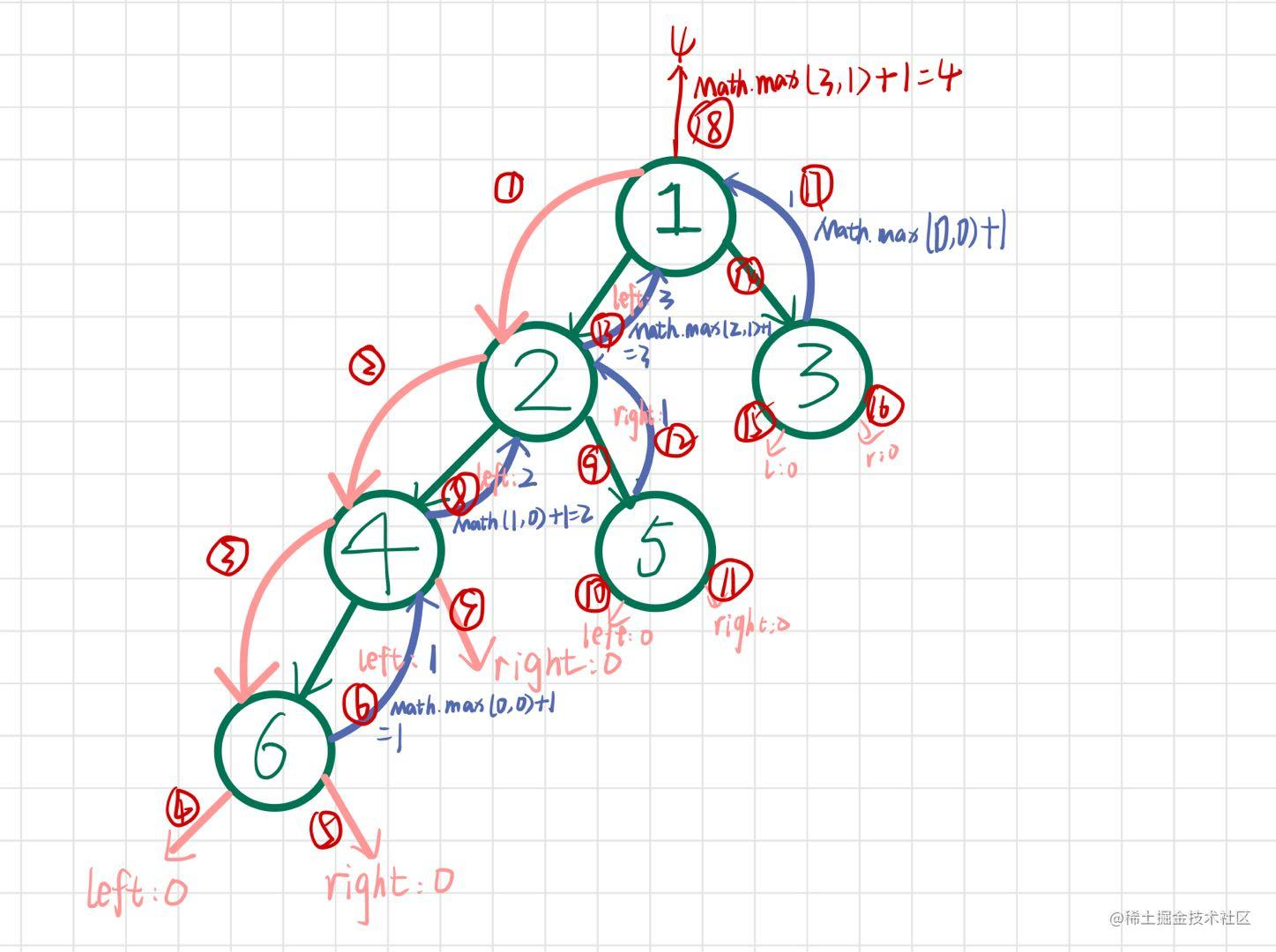``````var maxDepth = function(root) {
if(root == null) return 0;
//左子树的深度
let left = maxDepth(root.left);
//右子树的深度
let right = maxDepth(root.right);
//加上它本身
let res = Math.max(left,right) +1;
//返回结果
return res
};

• 226. 翻转二叉树 - 力扣（LeetCode）
• 难度：简单
• 这个题跟上面的题其实差不多的，只要你搞懂了是怎么去递归，然后改一改代码就好了
• 这道题解题思路就非常明确了：以递归的方式，遍历树中的每一个结点，并将每一个结点的左右孩子进行交换
``````var invertTree = function(root) {
if(!root) return null;
//交换常用写法，用temp变量暂存
let temp = root.left;
root.left = root.right;
root.right = temp;
invertTree(root.left);
invertTree(root.right);
return root
};

• 101. 对称二叉树 - 力扣（LeetCode）
• 难度：简单
• 这道题跟上面那一道记得要区分好；首先我们要搞清楚，究竟是怎么样的对称，和翻转的区别是什么
• 左右子树的根节点是否相等
• 左右子树是否镜像相等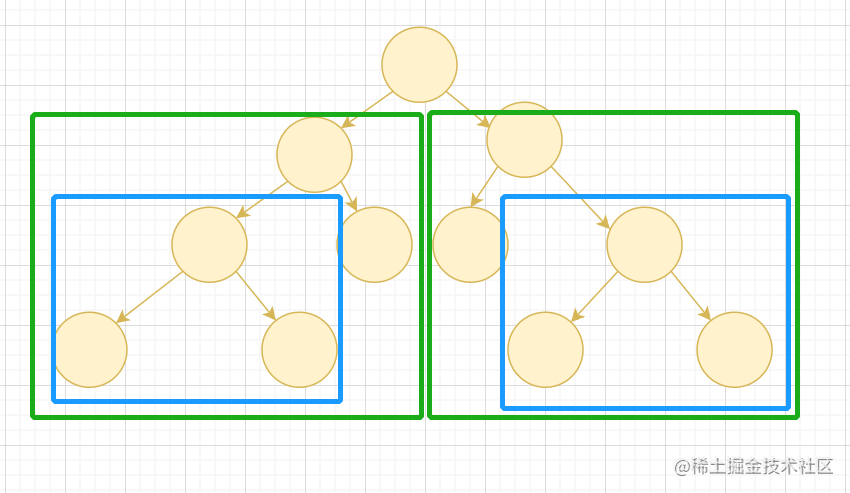• 思路：
• 以根节点作为起点
• 比较root1的左节点和root2的右节点
• 比较root1的右节点和root2的左节点
• 相等返回true，不等返回false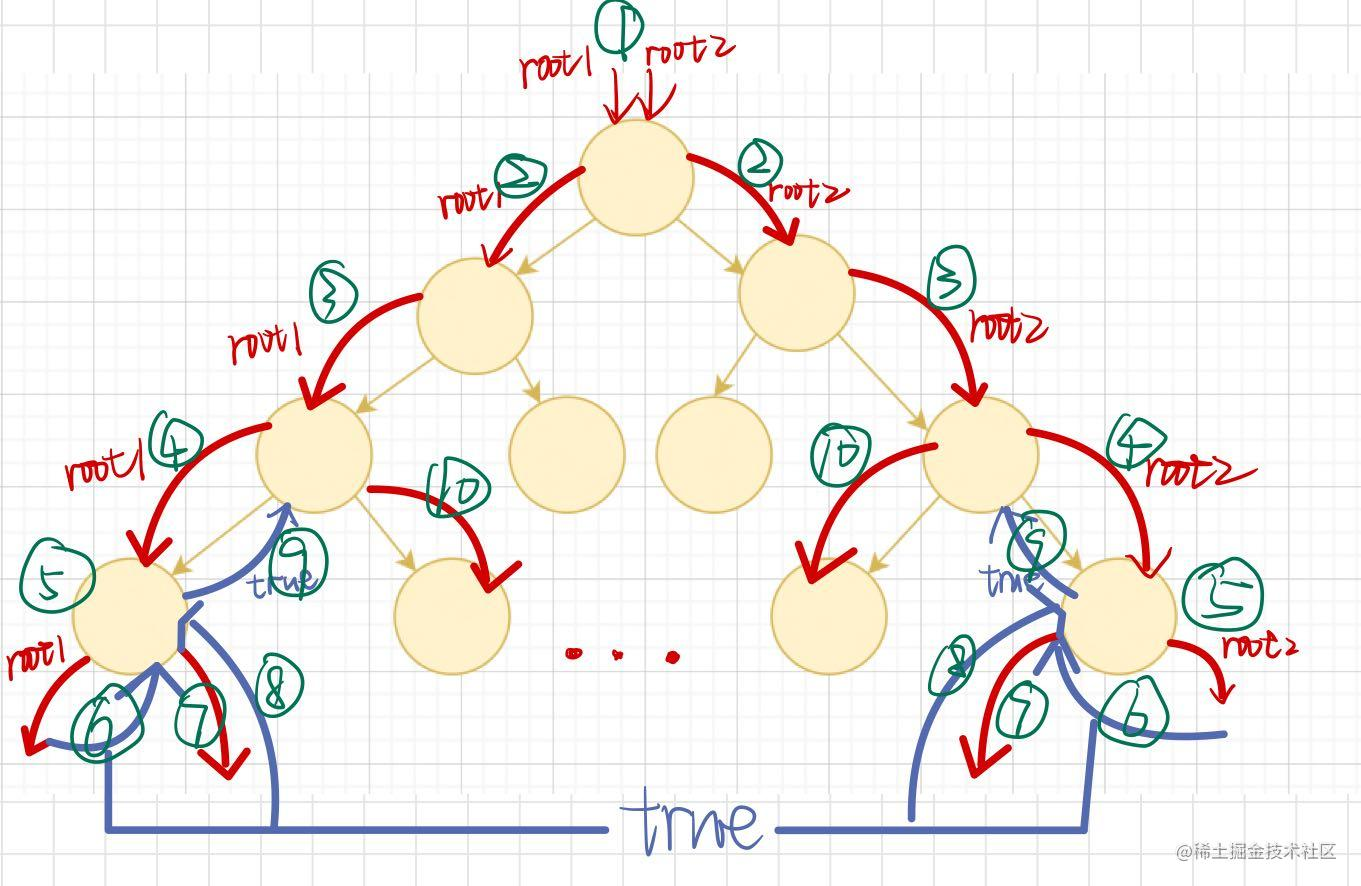``````var isSymmetric = function(root){
return isMirror(root,root)
};
const isMirror = function(root1, root2) {
//同时为空的时候肯等是对称的
if(!root1 && !root2) return true
//这里只剩下两种情况，要么root1为空，要么root2为空，那么肯等不对称
if(!root1 || !root2) return false
//比较节点的值
if(root1.val != root2.val) return false
//比较root1的左节点和root2的右节点 比较root1的右节点和root2的左节点
return isMirror(root1.left, root2.right) && isMirror(root1.right, root2.left)
}

• 236. 二叉树的最近公共祖先 - 力扣（LeetCode）
• 难度： 中等
• 不要被难度中等吓到，它依旧是很经典的题目，我们也依旧上我们的递归大法，考虑每一个节点需要做什么操作即可
• 我们分成三种情况
• p和q分别在根节点两侧，那么最近公共祖先就是根节点
• p和q都在左侧，则最先公共节点在左侧
• p和q都在右侧，则最先公共节点在右侧
• 思路：
• 如果树为空树或者p,q中任一节点为根节点，那么p,q的最近公共节点为根节点
• 如果树不为空且p,q为非根节点，则递归遍历左右子树，获取左右子树的最近公共祖先
• 我们由上面三种情况分别进行判断
• `left==null`则说明p,q都不在左子树，那么返回右子树结果
• `right==nul`l则说明p,q都不在右子树，那么返回左子树结果
• 若左边不为空，右边不为空，则说明该节点为他们的最近公共节点(因为我们是递归实现的，那么第一个找到的公共节点一定为他们最近公共节点)，那么返回该节点即可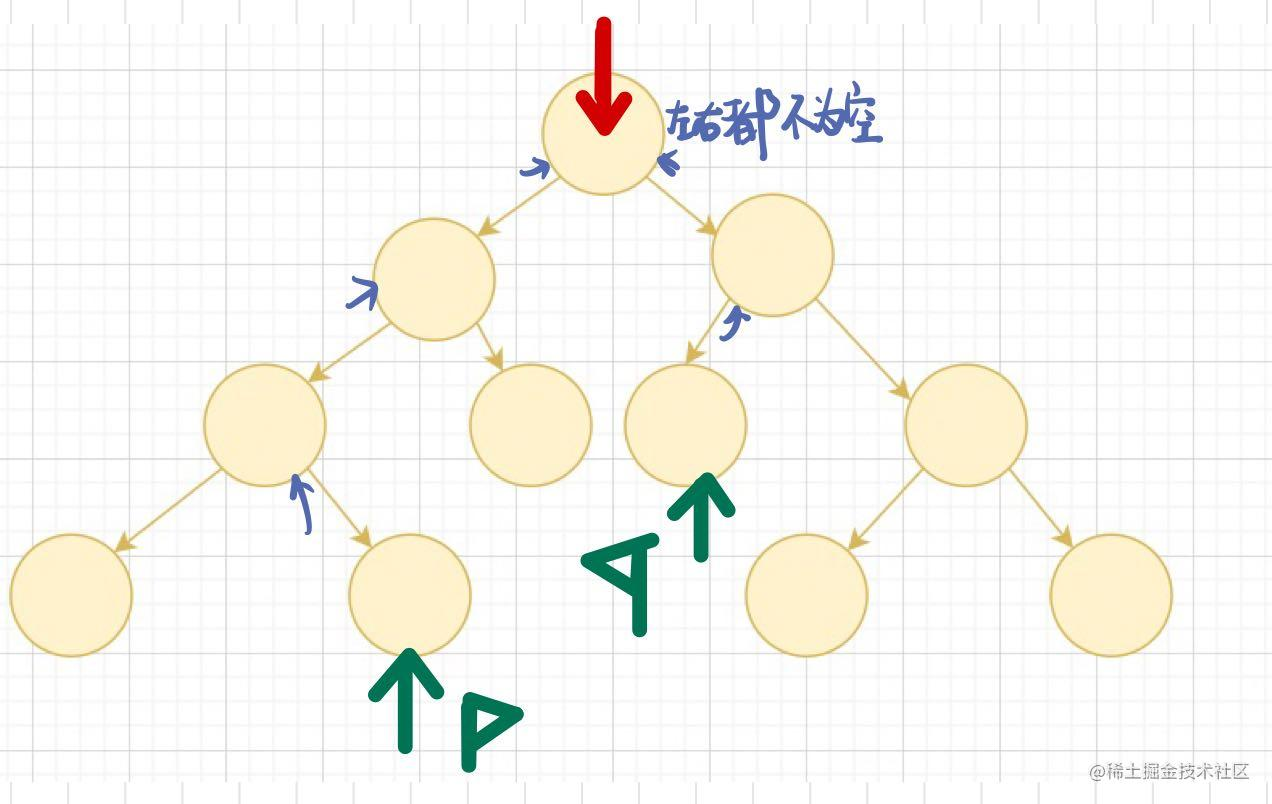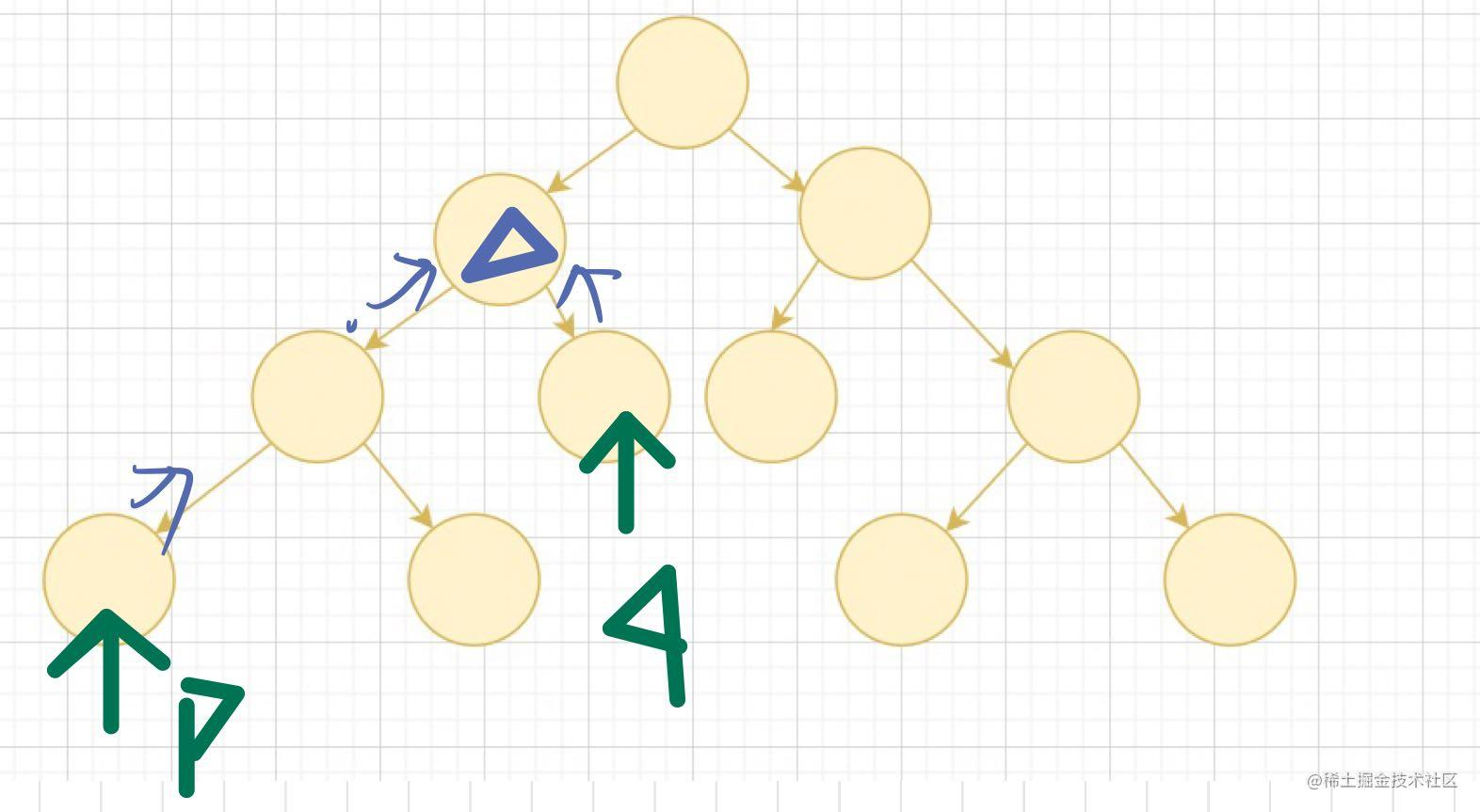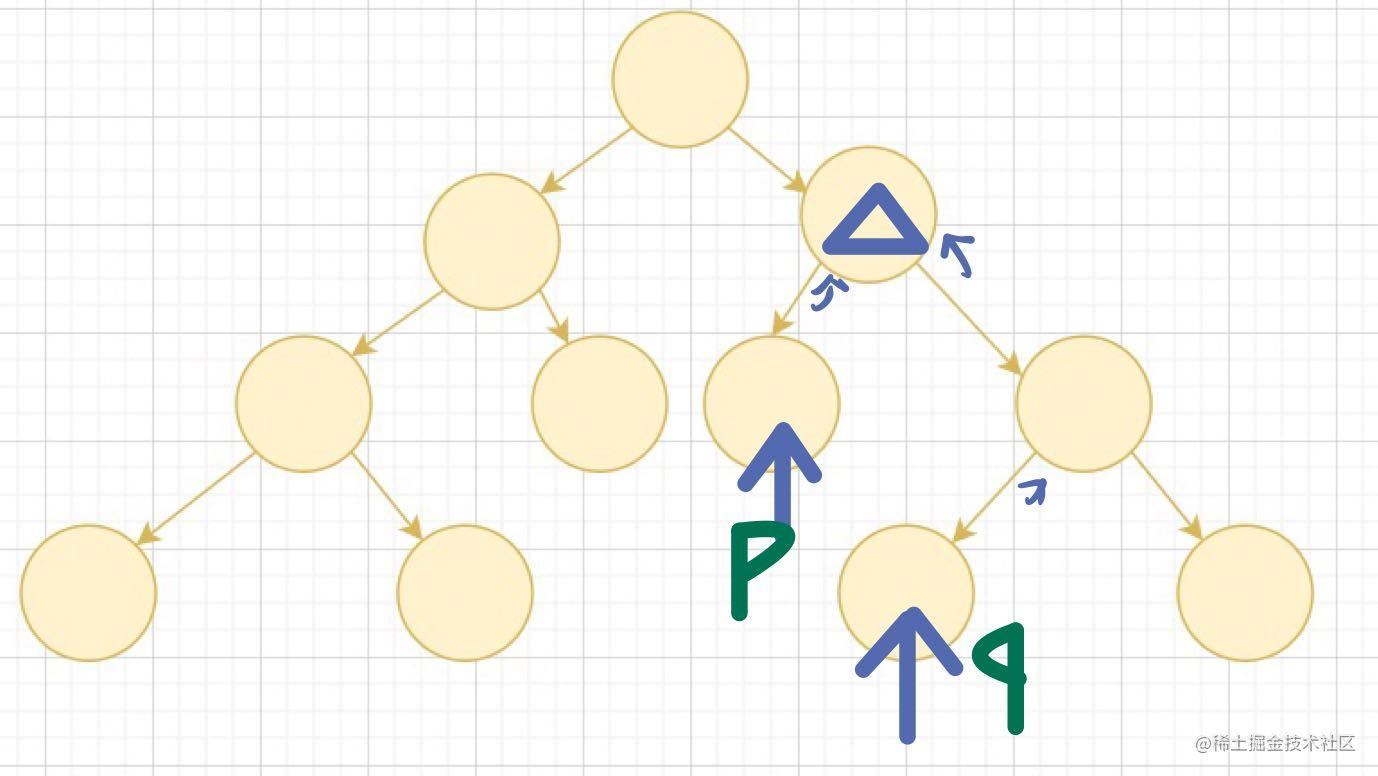``````var lowestCommonAncestor = function(root, p, q) {
//树为空，p,q在根节点上的情况全都直接返回根节点
if(!root || root == p || root ==q) return root;
const left = lowestCommonAncestor(root.left,p,q);
const right = lowestCommonAncestor(root.right,p,q);
if(left == null) return right; //说明p,q都不在左子树，那么返回右子树结果
if(right == null ) return left;//说明p,q都不在右子树，那么返回左子树结果
return root ;//说明p,q在根节点的两侧，返回根节点
};

## 二、二叉搜索树

• 首先我们先了解一下什么是二叉搜索树
• 二叉搜索树也叫排序二叉树，二叉查找树，简称BST
• 二叉搜索树指的是一颗空树或者其左子树上的所有节点的数据域都小于等于根节点的数据域，右子树所有结点的数据域都大于等于根节点的数据域
• 先来一道题巩固一下定义
• 98. 验证二叉搜索树 - 力扣（LeetCode）
• 难度：中等
• 我们上面说了两种情况，那么就直接分析这两种情况
• 空树：直接返回`true`
• 非空树：需要递归地对非空树中的左右子树进行遍历，检验每棵子树中是否都满足 `左 < 根 < 右` 这样的关系
``````var isValidBST = function(root) {
//初始化最大值和最小值
return dfs(root, -Infinity, Infinity)
};
const dfs = function(root,min,max) {
//满足空树条件，直接返回true即可
if(!root) return true;
//如果该右节点不符合右孩子不大于，或者左孩子不小于根节点，则不合法
if(root.val <= min || root.val >= max) return false
//每颗子树都要满足
return dfs(root.left, min, root.val) && dfs(root.right, root.val, max);
}

``````var searchBST = function(root, val) {
if(!root) return null;
if(root.val == val) return root;
const left = searchBST(root.left, val);
const right = searchBST(root.right, val);
return left || right;
};

• 做完你一想，如果这样的做法就能解决，那为什么要说明是二叉搜索树，是不是有更好的做法呢，如何利用起搜索二叉树左小右大的特性
• 如果结点已经比目标值小了其实我们就可以不用再递归其左子树了，
• 同样，如果结点已经比目标值大了，我们就不用再递归其右子树了
• 所以我们给递归加上判断即可
• 那么查找的节点为空的时候就说明该树找不到目标节点
``````var searchBST = function(root, val) {
if(!root) return null; //查找失败，直接返回
//节点数值已经比目标数值大了，那么就只需要去查找左子树
if(root.val > val) return searchBST(root.left, val);
//节点数值已经比目标数值小了，那么久只需要去查找右子树
if(root.val < val) return searchBST(root.right, val);
return root
};

• 701. 二叉搜索树中的插入操作 - 力扣（LeetCode）
• 难度：中等
• 虽然难度变成了中等，然后代码思维跟上一道题基本是一样的，回顾一下上一道题空节点的位置刚好就可以放入目标节点
• 所以只需要从根结点开始，把我们希望插入的数据值和每一个结点作比较。若大于当前结点，则向右子树探索；若小于当前结点，则向左子树探索。最后找到的那个空位，就是它合理的栖身之所
``````var insertIntoBST = function(root, val) {
if(!root) {
//插入节点的栖身之地
root = new TreeNode(val)
return root;
}
if(root.val > val) root.left = insertIntoBST(root.left, val);
if(root.val < val ) root.right = insertIntoBST(root.right,val);
return root
};

• 450. 删除二叉搜索树中的节点 - 力扣（LeetCode）
• 难度：中等
• 这道题是基于第一道题的扩展，我们要删除节点，首先就要先找到该节点
• 如果找不到，则直接返回
• 如果找到后，他没有左右子树则直接删除即可
• 如果找到后，他只有一个非空子节点，那么直接让该子节点接替他位置即可
• 如果找到后，他两个子节点都非空，那我们为了不破坏BST的特性，只能去其左子树找到左子树的最大值替掉他，或者去其右子树找到最小的替调他（！所以有一些答案不唯一）
• 思路清晰之后，我们只需要一步一步实现即可
• 首先先找到该节点
``````var deleteNode = function(root, key) {
if(!root) return root;
if(root.val == key) {
//找到结点，等会来找删除操作
console.log('我找到你啦', root.val); //我找到你啦，对应key值
return root
}
if(root.val > key) root.left = deleteNode(root.left, key);
if(root.val < key) root.right = deleteNode(root.right, key);
return root
};
复制代码``````
• 没有左右子树,直接删除
`````` if(!root.left && !root.right) {
root = null
}
复制代码``````
• 只有一个非空节点
``````if(!root.left || !root.right) {
root = root.left ? root.left: root.right;
}
复制代码``````
• 两个节点都非空，我们先采取去其右子树找到最小的
``````  const mid = getMin(root.right); //找到右子树最小节点
root.right = deleteNode(root.right, mid.val); //删除该结点
mid.left = root.left; //让最小节点去替换root节点
mid.right = root.right;
root = mid;
//找到最小节点
const getMin = function(node) {
//只需要找其右子树的左子树到最下面
while(node.left != null) {
node = node.left;
}
return node
}
复制代码``````
• 一样的思路，我们还可以采用去左子树找其最大的数
`````` const maxNode = getMax(root.left);
root.left = deleteNode(root.left, maxNode.val);
maxNode.left = root.left;
maxNode.right = root.right;
root = maxNode;
const getMax = function(node) {
while(node.right != null) {
node = node.right;
}
return node
}
复制代码``````
• 把每一步的代码合起来就是最终代码啦，这道题主要就是你需要先考虑好可能会出现什么情况，然后想好每一种情况是什么样的需要什么的处理，然后就是归纳整理优化代码了（这里采用的是去左子树找最大的数）
``````var deleteNode = function(root, key) {
if(!root) return root;
if(root.val == key) {
if(!root.left && !root.right) {
root = null
}else if(!root.left || !root.right) {
root = root.left ? root.left: root.right;
}else {
const maxNode = getMax(root.left);
root.left = deleteNode(root.left, maxNode.val);
maxNode.left = root.left;
maxNode.right = root.right;
root = maxNode;
}
return root
}
if(root.val > key) root.left = deleteNode(root.left, key);
if(root.val < key) root.right = deleteNode(root.right, key);
return root
};
const getMax = function(node) {
while(node.right != null) {
node = node.right;
}
return node
}

## 三、平衡二叉树

• 平衡二叉树指的是任意结点左右子树高度差绝对值都不大于1二叉搜索树
• 直接上题巩固一下定义
• 110. 平衡二叉树 - 力扣（LeetCode）
• 难度：简单
• 需要满足三个条件
• 每个节点左子树为平衡二叉树
• 每个节点右子树也为平衡二叉树
• 每个节点的的左子树和右子树的高度差绝对值不超过1
• 求绝对值使用Math.abs()
• 高度可以直接使用我们前面做过的求深度算法，照搬就是了
``````var isBalanced = function(root) {
if(!root) return true; //空树是平衡二叉树也是搜索二叉树，直接返回true即可
//递归查找三个条件
return isBalanced(root.left) && isBalanced(root.right) && Math.abs(maxDepth(root.left) - maxDepth(root.right)) <=1
};
const maxDepth = function(root) {
if(!root) return 0;
return Math.max(maxDepth(root.left), maxDepth(root.right))+1
}

• 108. 将有序数组转换为二叉搜索树 - 力扣（LeetCode）
• 难度：简单
• 这道题看题目是二叉搜索树相关，但是详细看你会发现他要求的是转为高度平衡二叉树，也就是平衡二叉树啦
• 这道题还涉及了一个特性：二叉搜索树的中序遍历序列是有序的（因为左小右大），刚好跟我们题目给的有序数组对应，所以有序数组的中间数字应该为树的根节点，那么思路就很明显了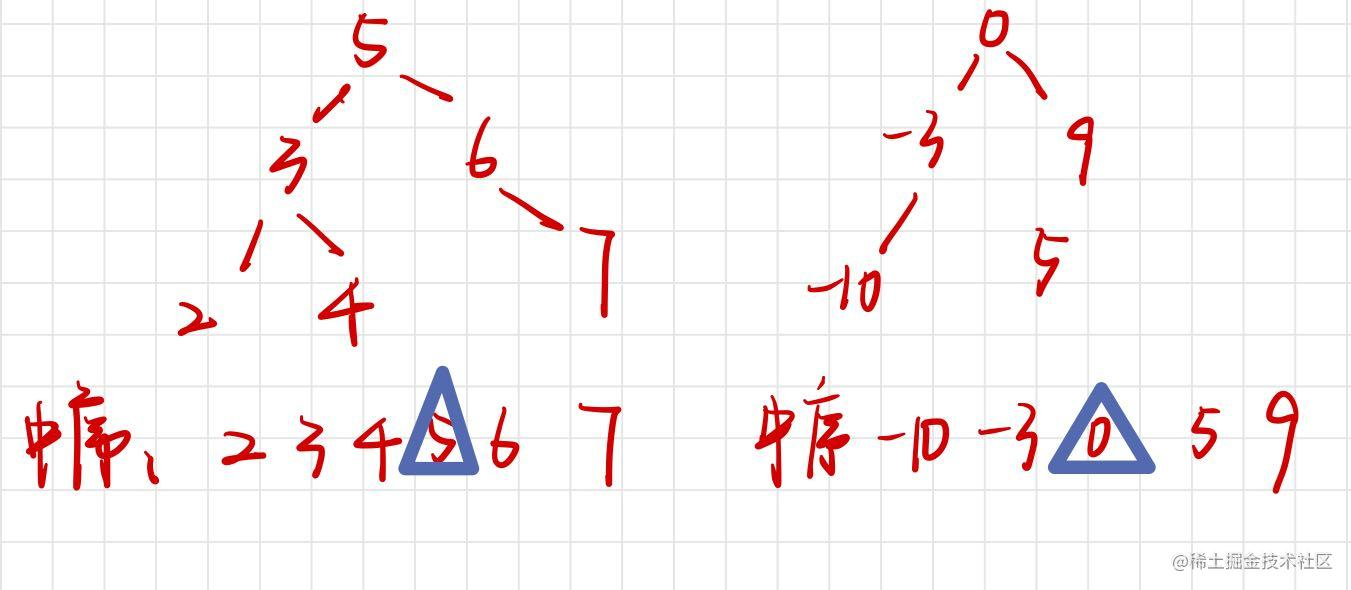• 我们先找到数组中间数字，创建一个新节点
• 左子树为该数组中间数字左边的数组进入递归
• 右子树为该数组中间数组右边的数组进入递归
• 如果数组为空的时候就返回null
``````var sortedArrayToBST = function(nums) {
//数组为空的时候返回空
if(!nums.length) return null
//找到中间结点
const middle = Math.floor(nums.length/2);
//new一个新节点
const root = new TreeNode(nums[middle]);
//采用slice以milddle为分割点去分割nums数组
root.left = sortedArrayToBST(nums.slice(0,middle));
root.right = sortedArrayToBST(nums.slice(middle+1));
return root
};

## 四、其他题（看到就写系列）

``````var connect = function(root) {
if(!root || !root.left) return null;
root.left.next = root.right;
connect(root.left);
connect(root.right);
return root
};

• 做完一看，不对，好像5和6没有连接起来，你得到的是这样的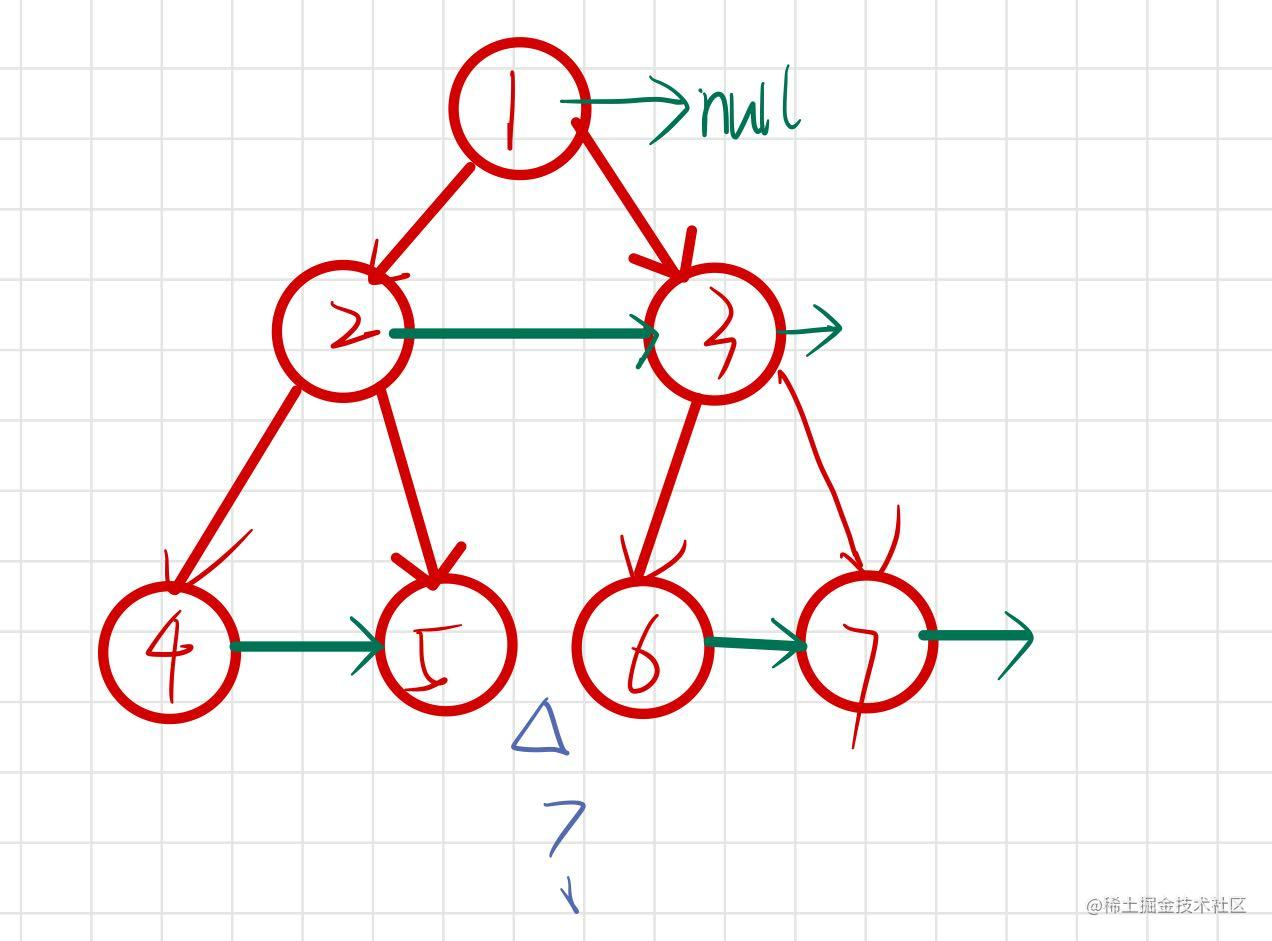• 仔细一想，我们这种解法好像不能解决不同父节点的连接，那么我们可以尝试着自己去定义需要连接的节点,以上图为例
• 4和5，6和7为同一父节点的应该相连
• 5和6为父节点为兄弟节点的应该相连
``````var connect = function(root) {
if(!root) return null;
traverse(root.left, root.right);
return root
};
const traverse = function(root1, root2) {
if(!root1 || !root2) return;
root1.next = root2;
//同一父节点
traverse(root1.left,root1.right);
traverse(root2.left,root2.right);
//跨越父节点
traverse(root1.right,root2.left);
}